// Strawberry Fields

# A Comparison of Quantum Algorithms for the Maximum Clique Problem

November 11, 2021We set out to compare the details of implementing a well known problem using the qubit gate model and boson sampling, to see the strengths and weaknesses of both approaches.

By Andy Haverly and Sonia Lopez Alarcon

Two versions of quantum computing receiving a lot of attention currently are qubit-based and continuous variable, taking the shape of the qubit gate model and boson sampling, respectively. While the qubits used in the qubit gate model are mostly based on the discrete values of the spin ½ particles, boson sampling is a form of quantum computing that uses the bosons’ position and momentum as continuous variables to be acted upon. Xanadu has created a successful boson sampler that uses photons and optical equipment to realize this model. Both of these models show promising results, but are fundamentally different in their design and their applications.

A direct comparison for solving a problem using the qubit gate model and boson sampling allows one to better understand the two approaches and the applications that each might be better suited for.

## The Maximum Clique Problem

The focus of this work is to use the maximum clique problem to examine the application development process in both models. The maximum clique problem was chosen because it is a well known NP-Hard  problem that can potentially be solved in both environments. In this case, these two environments are Qiskit and Strawberry Fields.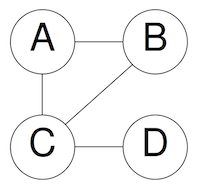A clique is a fully connected subgraph. The maximum clique problem is the task of finding the largest clique in a given graph. For example, in the picture above, the maximum clique of the graph is the subgraph with the vertices A, B, and C. This problem has applications in a number of fields, such as communications, social studies, marketing and social media.

## The qubit implementation: Grover’s Algorithm

Grover’s algorithm is a quantum algorithm that truly shows the potential speedups for quantum computing using the properties of superposition, entanglement, and phase kickback. Grover’s algorithm can search an unsorted list of N elements using O(sqrt(N)) steps, compared to the classical time complexity of O(N). The maximum amplitude of the probability for the solution states is found by iteratively increasing the amplitude of the solution states in a superposition of states .

Grover’s algorithm has three main components: initialization, the oracle, and the diffuser. The diffuser is standard for all implementations of Grover’s algorithm, but the initialization and the oracle were the partial focus of this work. Grover’s algorithm uses an oracle to determine if the selected state is a solution state. If it is, then it will have a higher chance of being measured after the diffuser is applied.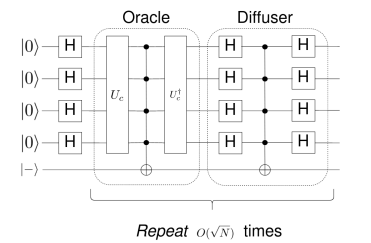## Using Grover’s Algorithm to Solve the Maximum Clique Problem

The implementation of this algorithm involved iteratively checking whether the number of existing edges in each possible subgraph (clique) was larger than a reference k, growing from 1 to the total number of possible edges in the full graph. The superposition state of all vertex/edges represented in the qubits allowed making this comparison in one single quantum comparison for each k. The implementation successfully identified the maximum cliques of the graphs that were tested .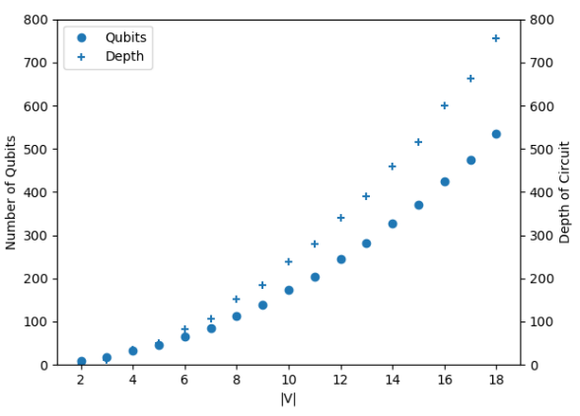Although the brief description above may seem straight forward, the actual quantum implementation grew in complexity quickly. The figure below depicts the implementation for a 3 vertex graph. The width (number of qubits) and depth (number of gate levels) can be used as metrics of this complexity, resulting in 18 qubits and 57 gate levels for a small 3 vertex case. For the 4 vertex case, 34 qubits and 98 gate levels were necessary, demonstrating that scalability is a challenge as the problem grows in size .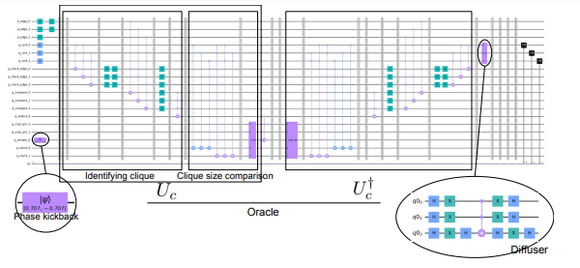## Boson Sampling: A Hybrid Implementation

Boson Sampling can be used to sample dense subgraphs from a graph . While this may produce subgraphs that are very similar to cliques, they are not guaranteed to be cliques, or even locally maximally dense subgraphs. However, following the steps of simulated annealing, a hybrid implementation can solve this problem by adding two steps: First, the least-dense vertices of the dense subgraph are removed to convert it into a clique. Then, the resulting clique is grown by adding fully connected vertices until there are no more possible vertices to add . These two additional steps are implemented classically, outside the boson sampling engine.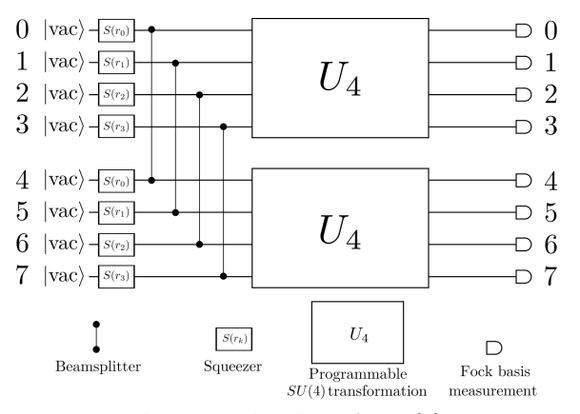The boson sampling step was implemented in Strawberry fields. The key component of this implementation is to map the graph’s adjacency matrix to the programmable four-mode rotation gates (SU(4) in the figure above) . This has two main limitations in currently available Xanadu hardware: the maximum size of the matrix is 4x4, and the graph has to be a bipartite graph due to the replicated structure of Xanadu’s X8 chip. This adjacency matrix is iteratively modified following the two classical steps described above.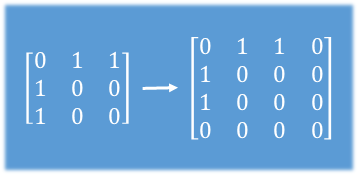One more factor to be determined is the mean photon number per mode. This value indicates how many photons the boson sampling device needs to create when given an exact number of modes. In this case, this number was determined using a trial and error strategy, with the mean the density of the graph as a starting point, until the squeezing amplitudes are not flagged as too low or too high by the Strawberry Fields engine.

## Qubit Gate vs Boson Sampling

The two implementations were able to provide the correct results for 3 and 4 vertex graphs. The boson sampling solution was implemented on the actual hardware provided by Xanadu, while the Qiskit implementation was simulated, due to the limited number of qubits available in the open hardware by IBM (15 qubits). Interestingly, these two different approaches had the same limiting graph size, in one case due to the maximum U4 transformations available in the X8 hardware by Xanadu, and in the other, due to the complexity of the circuit and memory requirements for the Qiskit simulation process.

The main difference between these two approaches was the complexity in the development process of the qubit-gate approach, which required weeks of work, and fine tuning with each graph size change. Strawberry Fields on the other hand, allowed for a straightforward mapping of the adjacency matrix to the U4 rotation blocks in the hardware available. It is worth noting, however, that as long as the graph is bipartite (as in this case), then boson sampling is ideally suited for this kind of problem, while in general boson sampling has serious limitations on its versatility. The gate-based approach, on the other hand, is much more versatile in nature, thanks to the almost infinite combinations of quantum gates that can potentially be included in the circuit description.

 K. Srinivasan, S. Satyajit, B. Behera, and P. Panigrahi, “Efficient quantum algorithm for solving travelling salesman problem: An ibm quantum experience,” 05 2018.

 L. K. Grover, “A fast quantum mechanical algorithm for database search,” Proceedings of the twenty-eighth annual ACM symposium on Theory of computing -STOC 96, 1996

 A. Haverly and S. Lopez. “Implementation of Grover’s Algorithm to solve the Maximum Clique Problem”. International Symposium on VLSI. July 2021

 U. Feige, G. Kortsarz, and D. Peleg, “The dense k-subgraph problem,” Algorithmica, vol. 29, p. 2001, 1999.

 X. Geng, J. Xu, J. Xiao, and L. Pan, “A simple simulated annealing algorithm for the maximum clique problem,” Information Sciences, vol. 177, no. 22, pp. 5064–5071, 2007.

J. M. Arrazola et al. “Quantum circuits with many photons on a programmable nanophotonic chip” Nature, March 2021.

Published on November 11, 2021